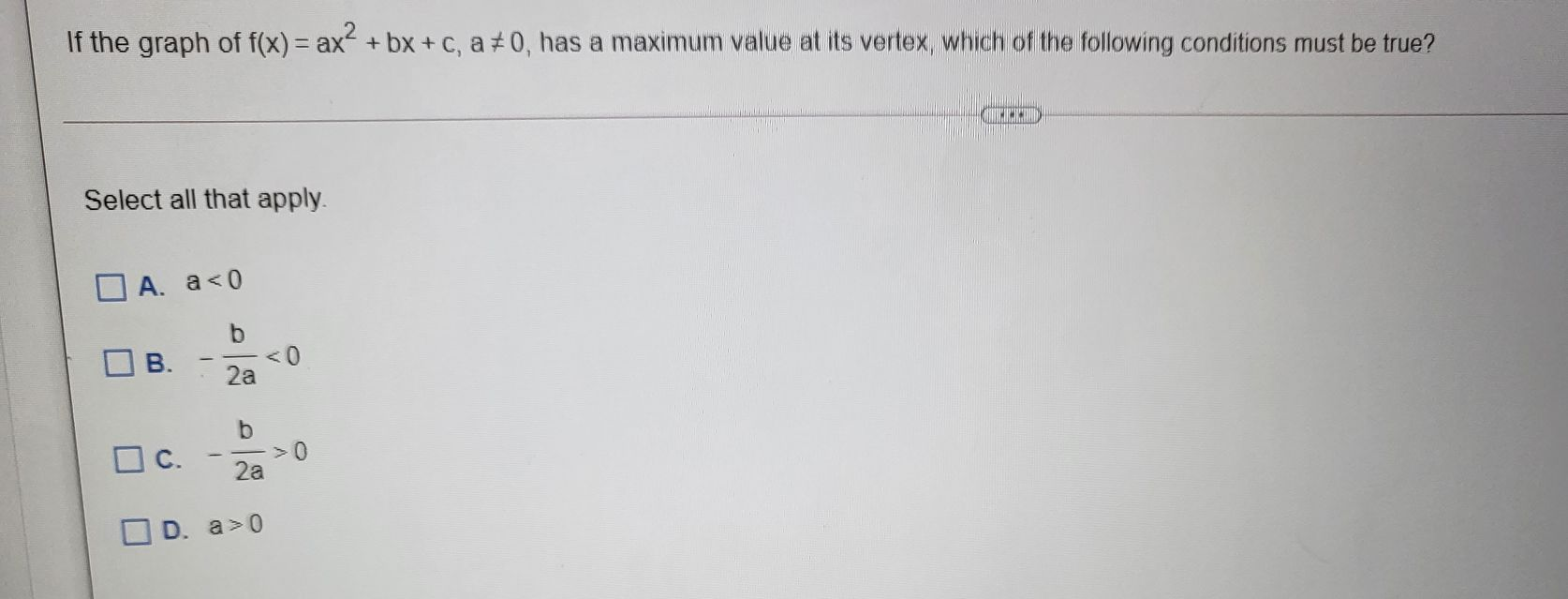### Still have math questions?

Algebra
QuestionIf the graph of $$f ( x ) = a x ^ { 2 } + b x + c , a \neq 0 ,$$ has a maximum value at its vertex, which of the following conditions must be true? Select all that apply.

$$\square$$ A. $$a < 0$$ B. $$- \frac { b } { 2 a } < 0$$

$$\square$$ C. $$- \frac { b } { 2 a } > 0$$

D. $$a > 0$$